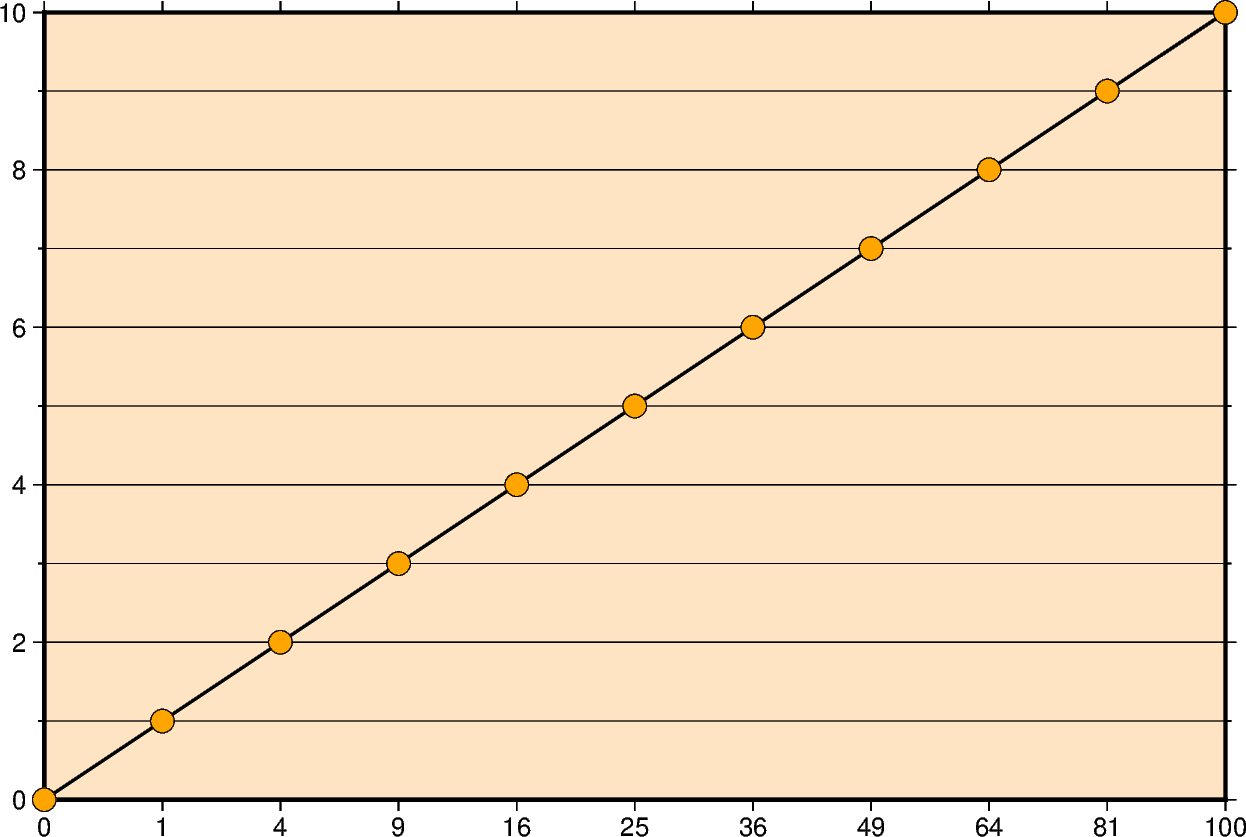# Cartesian power

Xwidth[ppvalue]/[height[ppvalue]]: Give the width of the figure and the optional argument height. The axis or axes with a logarithmic transformation requires p and the power transformation for that axis.Out:

```<IPython.core.display.Image object>
```

```import numpy as np
import pygmt

# Create a list of y values 0-10
yvalues = np.arange(0, 11)
# Create a list of x-values that are the square of the y-values
xvalues = yvalues**2

fig = pygmt.Figure()
fig.plot(
region=[0, 100, 0, 10],
# Set the power transformation of the x-axis, with a power of 0.5
projection="X15cp0.5/10c",
# Set the figures frame, color, and gridlines
frame=["WSne+givory", "xa1p", "ya2f1"],
# Set the line thickness to *thick*
# Use the default color *black* and the default style *solid*
pen="thick",
x=xvalues,
y=yvalues,
)
# Plot x,y values as points on the line
# Style of points is 0.2 cm circles, color is *green* with a *black* outline
# Points are not clipped if they go off the figure
fig.plot(x=xvalues, y=yvalues, style="c0.2c", color="green", no_clip=True, pen="black")
fig.show()
```

Total running time of the script: ( 0 minutes 0.951 seconds)

Gallery generated by Sphinx-Gallery